Search IntMath
Close

450+ Math Lessons written by Math Professors and Teachers

5 Million+ Students Helped Each Year

1200+ Articles Written by Math Educators and Enthusiasts

Simplifying and Teaching Math for Over 23 Years

# Is a 1×1 matrix a scalar?

By Murray Bourne, 26 Nov 2015

Hello! 🙂

I just had a problem and got stuck when i tried to multiply (A.B).C where A ,B and C are three matrices with dimensions 1x3, 3x1 and 3x1 respectively. I got the product of (A.B) with a 1x1 dimensional matrix.

The question is: can a 1x1 matrix be a scalar? So should I just stop and say that 1x1 and 3x1 can't be multiplied?

Or is the matrix  = 2 a scalar (for example)?

And continue multiplying the scalar (AB) with the matrix C?

This was an interesting question. First, let me explain the issue a bit.

## Multiplying matrices

We learn in the Multiplying Matrices section that we can multiply matrices with dimensions (m × n) and (n × p) (say), because the inner 2 numbers are the same (both n). The result will be a vector of dimension (m × p) (these are the outside 2 numbers).

Now, in Nour's example, her matrices A, B and C have dimensions 1x3, 3x1 and 3x1 respectively.

So let's invent some numbers to see what's happening.

Let's let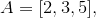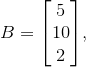and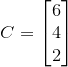Now we find (AB)C, which means "find AB first, then multiply the result by C".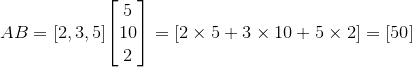Next,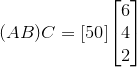The above line is Nour's dilemma. Are we allowed to multiply the above? We have the following situation:

(1 × 1) matrix × (3 × 1) matrix

According to what I said above, since the inside numbers (1 and 3) are different, then we can't multiply the matrices.

However, if we regard a (1 × 1) matrix as a scalar, we can multiply them just fine, and get the following result: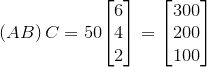Which is correct?

## Software solutions

Scientific Notebook gave me the following for the first step: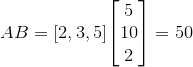That is, it regards a 1×1 matrix as a scalar. It was fine with the scalar times matrix step and gave the same as my second result above.

However, it choked (it refused to answer) when I tried to do it all in one go, like this: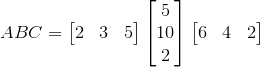Even giving it a hint (by putting brackets) didn't help: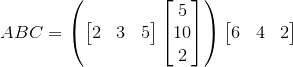Wolfram|Alpha regards the result of AB as a (1 × 1) matrix. Here's a screen shot: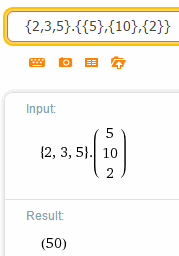It did not behave well and gave rather strange results when trying to do the whole thing. Here's a screen shot of its answer for ABC, where it regarded parts of the question as a vector, and other parts as a matrix: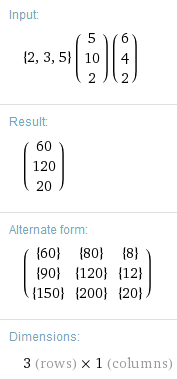SageMath (the free cloud-based computer algebra system) also regards the result of AB as a (1 × 1) matrix. It appropriately chokes when trying to do the whole thing. Here's a screen shot: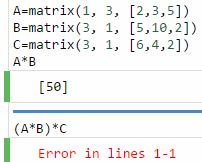## What do the forums say?

Here's one conversation on Stackexchange: Are one-by-one matrices equivalent to scalars?

This next one has a contradictory conclusion: Is a one by one matrix just a number scalar?

And there's more enlightenment here on this Google+ discussion.

## What I told Nour

My feel is that your statement "So i will just stop and say that 1x1 and 3x1 can't be multiplied" is correct. While the outer numbers are the same, the inner ones are not, so you can't multiply them.

What do you think?

## Conclusion

Mathematics is not always a consistently behaved beast, despite what many text books say.

### 10 Comments on “Is a 1×1 matrix a scalar?”

1. Nathan says:

I agree with the (currently second place) response to the first stackexchange post: it is can be treated as a scalar because we treat the dot product as a scalar, which is the result of a [1xN]*[Nx1] multiplication. In fact, I believe a 1x1 matrix like this must, by definition, be the dot product of two vectors, and so all those considerations should apply.

2. Tomas Garza says:

Murray says: "Mathematics is not always a consistently behaved beast, despite what many text books say". I think this is a rather harsh statement, especially if we look at the situation under consideration.

In my view, one has to be clear as to what kind of things one is dealing with. For example, when we talk about "the algebra of real numbers", the only valid statements are those which deal with real numbers. We know then that "the set of real numbers is closed under multiplication", etc. Now, if one asks, for example, what are the n roots of the real number 1, this question has no meaning inside the algebra of the real numbers, and that is the reason why one has to go one step further and enter the algebra of complex numbers.

This goes for things such as vectors and matrices. The vector  is a vector with one component, and it is not a number. The matrix  which has one row and one column is not a number.
This also goes for sets of numbers: there is a fundamental difference between the number 1 and the set which comprises only one element, namely the number 1.

In the special situation brought about by Nour, I would simply say: it is allright to multiply a vector or a matrix by a scalar (i.e., a real number); the algebra of vectors or matrices has that operation clearly defined. But if you have a matrix, say, of dimensions 1 x 1, this is not a number and you cannot multiply this by a matrix that doesn't have the right dimensions.

3. Murray says:

My "harsh" statement was to trigger some thinking about a common myth (that we are all told) - that mathematics is some kind of perfectly consistent system. It mostly is, but not always. I should have included these links with my statement:

Is 0 a natural number?

Which is the correct graph of arccot x?

Is cube root the same as raising to power 1/3?

Towards more meaningful math notation

4. Rene Grothmann says:

The problem is that matrix multiplication is not the same as multiplication of a scalar with a matrix or vector. We write A*B and c*B in the same way, but we should not. We should write A*B and cB.

Moreover, if we allow the multiplication in question, we give up (A*B)*C=A*(B*C) for matrix multiplication. We don't want to do this.

5. Murray says:

@Rene: Actually, I don't agree we should write matrix multiplication with a * operator. It should have none, like AB, as should a scalar by a matrix, cB.

6. Alan Cooper says:

I don't think there's any notation that we "should" use, but I guess one thing we should NOT do is combine a convention where we use the same symbol (or none) both for matrix multiplication and for multiplication of matrices by scalars AND also identify a matrix [c] with the scalar c.(Unless we also adopt as a third convention that a scalar multiplies a row vector only from the left and a column vector only from the right)

If I wanted to have a separate operator symbol for matrix multiplication, then I'd probably use the same little 'o' that we often use for composition of functions, since that's what matrix multiplication really is.(Though I agree with one of the commenters in the posts you link to that this all really depends on what you think the meaning of "is" is.)

Although the consistency of mathematics is unprovable, I don't think there are any identified inconsistencies so far. What we are looking at here is an inconsistency in the way (some) mathematicians write about mathematics rather than in the subject matter itself.

7. Murray says:

@Alan: I should be more careful to rant about inconsistent math notation in particular, rather than mathematics in general.

I don't know that I agree with your first sentence - I think in this case we should agree on a consistent notation. That was the dilemma the student had - do we write it as a matrix, or as a scalar? It makes a difference regarding what we can do next.

8. Alan Cooper says:

..and I should be more careful not to quibble unnecessarily.

I do agree that we should try to agree on a consistent notation - and also think that it should be as consistent as possible with what is done most often in practice.

But consistency requires that we not combine dropping the operator with identifying a 1x1 matrix as a scalar.

One solution is to use simple juxtaposition both for matrix and scalar multiplication(ie multiplication of a matrix by a scalar number (*)) but then NOT to identify [c] with c.

Another is to identify [c] with c but use an explicit operator symbol for the case of matrix multiplication (I like the little 'o' even though I can't remember how to type it here, and even a dot would suffice since it's also a generalization of the inner product, but I'm not so keen on * because it is often used in computer languages for simple numerical multiplication and also in mathematics for convolution).

A commenter on one of the other posts you link to noted that the product of a scalar number times a matrix is more consistent with the outer or tensor product than the inner, dot, or "scalar" product.
(*- Above I used "scalar multiplication" perhaps inadvisedly to refer to multiplication by a scalar. But how many students are confused by the fact that, in dimensions greater than one, a scalar multiple is not a scalar product? or do they even notice?)

9. Les says:

In Maple 2015 (Maplesoft) matrices and vectors can be multiplied using the period symbol (.), another option is to use the element-wise operator (*~).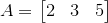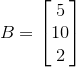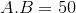50*B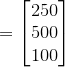A.B.C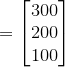But a 1×1 multiplied by a 3×1 is undefined.

With element-wise operator:

A*~B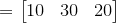A*~B*~C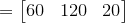10. Murray says:

Thanks for the input, Les - that clarifies it a lot.

Good on you for using the math entry system. Sometimes it's a challenge - WordPress was changing some things (the tilde) and the LaTeX image was not quite what you meant, so I edited it a bit for you.

I hope it now reads as you intended.

### Comment Preview

HTML: You can use simple tags like <b>, <a href="...">, etc.

To enter math, you can can either:

1. Use simple calculator-like input in the following format (surround your math in backticks, or qq on tablet or phone):
a^2 = sqrt(b^2 + c^2)
(See more on ASCIIMath syntax); or
2. Use simple LaTeX in the following format. Surround your math with $$ and $$.
$$\int g dx = \sqrt{\frac{a}{b}}$$
(This is standard simple LaTeX.)

NOTE: You can mix both types of math entry in your comment.